# JP4390646B2 - Spread spectrum clock generator and modulation method thereof - Google Patents

## Info

Publication number
JP4390646B2
JP4390646B2 JP2004203320A JP2004203320A JP4390646B2 JP 4390646 B2 JP4390646 B2 JP 4390646B2 JP 2004203320 A JP2004203320 A JP 2004203320A JP 2004203320 A JP2004203320 A JP 2004203320A JP 4390646 B2 JP4390646 B2 JP 4390646B2
Authority
JP
Japan
Prior art keywords
modulation
circuit
signal
oscillation
control
Prior art date
Legal status (The legal status is an assumption and is not a legal conclusion. Google has not performed a legal analysis and makes no representation as to the accuracy of the status listed.)
Active
Application number
JP2004203320A
Other languages
Japanese (ja)
Other versions
JP2006024110A (en
Inventor

Original Assignee
Ｎｅｃエレクトロニクス株式会社
Priority date (The priority date is an assumption and is not a legal conclusion. Google has not performed a legal analysis and makes no representation as to the accuracy of the date listed.)
Filing date
Publication date
Application filed by Ｎｅｃエレクトロニクス株式会社 filed Critical Ｎｅｃエレクトロニクス株式会社
Priority to JP2004203320A priority Critical patent/JP4390646B2/en
Publication of JP2006024110A publication Critical patent/JP2006024110A/en
Application granted granted Critical
Publication of JP4390646B2 publication Critical patent/JP4390646B2/en
Active legal-status Critical Current
Anticipated expiration legal-status Critical

• 230000000051 modifying Effects 0.000 title claims description 198
• 238000001228 spectrum Methods 0.000 title claims description 32
• 230000007274 generation of a signal involved in cell-cell signaling Effects 0.000 claims description 17
• 239000004065 semiconductor Substances 0.000 claims description 8
• 230000000694 effects Effects 0.000 description 8
• 238000006243 chemical reaction Methods 0.000 description 3

## Images

•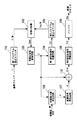•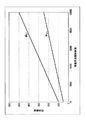•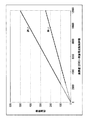•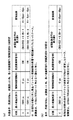•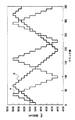•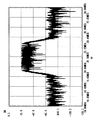•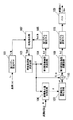•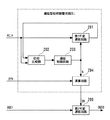•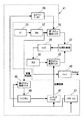•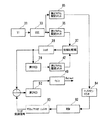•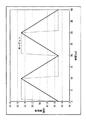•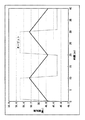•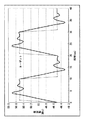•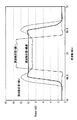•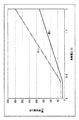## Classifications

• HELECTRICITY
• H03BASIC ELECTRONIC CIRCUITRY
• H03LAUTOMATIC CONTROL, STARTING, SYNCHRONISATION, OR STABILISATION OF GENERATORS OF ELECTRONIC OSCILLATIONS OR PULSES
• H03L7/00Automatic control of frequency or phase; Synchronisation
• H03L7/06Automatic control of frequency or phase; Synchronisation using a reference signal applied to a frequency- or phase-locked loop
• H03L7/08Details of the phase-locked loop
• H03L7/0805Details of the phase-locked loop the loop being adapted to provide an additional control signal for use outside the loop
• HELECTRICITY
• H03BASIC ELECTRONIC CIRCUITRY
• H03LAUTOMATIC CONTROL, STARTING, SYNCHRONISATION, OR STABILISATION OF GENERATORS OF ELECTRONIC OSCILLATIONS OR PULSES
• H03L7/00Automatic control of frequency or phase; Synchronisation
• H03L7/06Automatic control of frequency or phase; Synchronisation using a reference signal applied to a frequency- or phase-locked loop
• H03L7/08Details of the phase-locked loop
• H03L7/081Details of the phase-locked loop provided with an additional controlled phase shifter
• H03L7/0812Details of the phase-locked loop provided with an additional controlled phase shifter and where no voltage or current controlled oscillator is used
• H03L7/0816Details of the phase-locked loop provided with an additional controlled phase shifter and where no voltage or current controlled oscillator is used the controlled phase shifter and the frequency- or phase-detection arrangement being connected to a common input
• HELECTRICITY
• H03BASIC ELECTRONIC CIRCUITRY
• H03LAUTOMATIC CONTROL, STARTING, SYNCHRONISATION, OR STABILISATION OF GENERATORS OF ELECTRONIC OSCILLATIONS OR PULSES
• H03L7/00Automatic control of frequency or phase; Synchronisation
• H03L7/06Automatic control of frequency or phase; Synchronisation using a reference signal applied to a frequency- or phase-locked loop
• H03L7/08Details of the phase-locked loop
• H03L7/099Details of the phase-locked loop concerning mainly the controlled oscillator of the loop
• H03L7/0995Details of the phase-locked loop concerning mainly the controlled oscillator of the loop the oscillator comprising a ring oscillator
• H03L7/0997Controlling the number of delay elements connected in series in the ring oscillator

## Description

The present invention relates to an apparatus and a method for clock frequency spread (also referred to as “SSCG”) for reducing EMI (Electromagnetic Interference) of a semiconductor integrated circuit.

With the progress of high-speed and high-performance semiconductor integrated circuits, so-called EMI countermeasures that keep radiated noise within regulation values have become much more difficult. Even if countermeasure parts such as an EMI filter and a decoupling capacitor are inserted into the printed circuit board, the regulation value may not be cleared.

Also, inserting EMI countermeasure components results in increased occupancy area and cost. Among many clock-synchronized semiconductor integrated circuits, attention is focused on SSCG technology that modulates the clock frequency to reduce the peak spectrum.

FIG. 9 is a diagram illustrating a configuration described in Patent Document 1. In FIG.

Y131 is a piezoelectric crystal used for the oscillation circuit 32, and generates a stable clock pulse train or an unmodulated clock signal. The first program setting counter 35 divides (divides) the unmodulated clock signal by an integer (M). A clock signal from a voltage controlled oscillator 39 (VCO) that varies the oscillation frequency based on the voltage from the filter 38 is input to the buffer 40, and an output clock is output from the buffer 40. The frequency of the clock signal is proportional to the input voltage from the phase comparator 37 and the filter 38. The output clock signal from the VCO 39 is input to the second program setting counter 42, and the clock signal from the VCO 39 is divided (divided) by an integer (N) and supplied to the phase comparator 37.

The phase comparator 37 and the filter 38 generate an analog signal proportional to the phase error between the first program setting counter 35 and the second program setting counter 42, and the clock signal from the buffer 40 generates the oscillation frequency time (N / M )be equivalent to. The spread spectrum modulation is performed using a spread spectrum modulation means 41 that changes M and N as a function of time. The third program setting counter 45 divides the output of the oscillation circuit 32 by an integer (I) that sets a rate at which M and N change or a modulation frequency.

The first and second look-up tables 46, 47 are M and N tabulated values that modulate the output clock signal frequency, respectively.

Up / down counter 49 is used to indicate the next entry in the lookup table. The serial link 51 is used to modify the modulation characteristics by programming different values into a program setting counter or look-up table.

FIG. 10 is a diagram showing another configuration described in Patent Document 1. In FIG. The spread spectrum modulation is performed by analog modulation signals output from the second VCO 52 and the digital / analog converter 83 (DAC).

The second VCO 52 generates the same clock as the first VCO 39 when there is no modulation. The second VCO 52 generates an expanded spectrum clock output signal in response to the analog modulation.

The modulation is performed by the ROM 82 that stores the modulation amplitude value sent to the digital / analog converter 83. The count output of the up / down counter 84 is used as an address of the ROM 82. The third program setting counter 85 sets the modulation frequency.

JP-A-7-235862 (FIGS. 6 and 9)

In the conventional configuration described above,
・ Manufacturing variation of semiconductor integrated circuits,
-There is a problem that the desired modulation degree cannot be obtained depending on the usage environment such as power supply voltage, ambient temperature, and usage frequency.

When the modulation degree is small, the EMI reduction effect is reduced. Conversely, when the modulation degree is large, the maximum frequency of the clock becomes high, and the circuit that supplies the clock may malfunction.

In the semiconductor integrated circuit, the characteristics of transistors, resistors, and capacitors that are configured vary depending on manufacturing variations, power supply voltage, and operating temperature. For this reason, the characteristics of the filter and the VCO change.

In the configuration of FIG. 9, the modulation clock is generated by changing the values of M and N. That is, the modulation clock signal is generated by making the VCO frequency respond to different frequencies. FIG. 11 shows an example of the frequency transition when the frequency response characteristic including the filter and the VCO characteristic and the switching time between M and N are designed to be optimal in design.

As described above, when the characteristics of the filter and the VCO change and the frequency response speed is slow, as shown in FIG. 12, the target frequency is switched before the modulation clock frequency becomes the target frequency. The degree of modulation is reduced. Since the EMI reduction effect tends to increase as the modulation degree increases, in the case of FIG. 12, the modulation degree is smaller than the expected modulation degree, and the EMI reduction effect decreases.

On the contrary, when the frequency response speed is high, as shown in FIG. 13, a state exceeding the target frequency and a state stable at the target frequency occur. If a clock that exceeds the operating frequency of the circuit that supplies the clock is supplied, it may cause a malfunction. Further, when the frequency is stagnated, the effect of spectral dispersion is reduced, and the EMI reduction effect is reduced.

FIG. 14 is a diagram showing an image of the spectrum in the states of FIGS. 11, 12, and 13. Even if the frequency response characteristics are the same, if the target clock frequency is different, the same phenomenon as when the frequency response characteristics change occurs.

When the clock frequency is high, it is equivalent to the case where the frequency response characteristic is slow, and when the clock frequency is low, it is equivalent to the case where the frequency response characteristic is fast.

In the case of the configuration shown in FIG. 10, a modulation clock is generated from the second VCO 52 that inputs a value obtained by adding the analog modulation signal from the digital-analog converter 83 to the output voltage from the filter 38 as a control voltage. The period is a factor that determines the modulation period and the amplitude determines the degree of modulation.

In the case of the configuration shown in FIG. 10, as described above, the characteristics of the VCO change due to variations in manufacturing, power supply voltage, and use temperature. An example of VCO control voltage and output frequency characteristics under two conditions is shown in FIG.

When an arbitrary frequency is output from the VCO, the control voltage (output voltage from the filter 38) varies depending on manufacturing variations and use conditions. When an analog modulation signal is added to the output voltage from the filter 38 to generate a spread spectrum clock, even if the amplitude of the analog modulation signal is constant, the output voltage from the filter 38 is different. Is not constant.

The same phenomenon occurs when the target frequency is different.

Accordingly, an object of the present invention is to provide a spread spectrum clock generation circuit and method capable of supplying a frequency modulation clock having a desired modulation degree even if manufacturing variations, power supply voltage, use temperature, and use frequency change. .

Another object of the present invention is to provide a spread spectrum clock generation circuit and method that prevent a reduction in the effect of EMI reduction.

Another object of the present invention is to provide a spread spectrum clock generation circuit and method for preventing malfunction of a circuit to which a clock is supplied.

Another object of the present invention is to provide a spread spectrum clock generation circuit and method that reduce the number of components for EMI countermeasures.

The invention disclosed in the present application is generally configured as follows in order to achieve the above object.

A spread spectrum clock generation circuit according to one aspect of the present invention includes a delay control type oscillation circuit that variably controls an oscillation period at equal intervals based on a control signal, and a minimum oscillation period of the delay control type oscillation circuit. A modulation maximum value determining circuit for determining a maximum value of a modulation signal from a value converted by a control period interval of an oscillation period and an oscillation frequency control value of the delay control type oscillation circuit; and the modulation maximum value determining circuit from the modulation maximum value determining circuit A modulation signal generation circuit that receives a maximum value and generates a modulation control signal within the modulation maximum value, and an addition value of the oscillation frequency control value and the modulation control signal is added to the delay control type oscillation circuit A frequency-modulated clock signal is output from the delay control type oscillation circuit provided as the control signal. In the present invention, the value obtained by converting the minimum oscillation period of the delay control type oscillation circuit by the control period interval of the oscillation period is constant even when the delay value of the element changes due to manufacturing variations or use conditions, The delay control type oscillation circuit outputs a frequency-modulated clock signal having a constant modulation degree.

A spread spectrum clock generation circuit according to another aspect of the present invention includes first and second delay control type oscillation circuits that vary an oscillation period at a predetermined control period interval according to a control signal, and the first delay. A phase comparator that compares the phase of the clock signal obtained by dividing the output clock signal of the control type oscillation circuit with the clock signal obtained by dividing the reference clock signal, and frequency control based on the phase comparison result of the phase comparator. A control circuit that generates a signal and supplies the first delay control type oscillation circuit as the control signal, a predetermined value, the frequency control signal, and a given modulation degree setting signal. A modulation maximum value determination circuit for determining a value; a modulation signal generation circuit for receiving the modulation maximum value from the modulation maximum value determination circuit and generating a modulation control signal within the modulation maximum value; The second delay control type oscillation circuit receives, as a control signal, a value obtained by adding the modulation control signal from the modulation signal generation circuit and the frequency control signal from the control circuit, and a frequency-modulated clock signal Is output. In the present invention, the predetermined value in the modulation maximum value determination circuit is a value obtained by converting the minimum oscillation period of the oscillation circuit into a control period interval. Alternatively, the minimum value of the frequency control signal is a value obtained by converting the minimum oscillation period of the oscillation circuit by the control period interval.

A spread spectrum clock generation method according to another aspect of the present invention includes a value obtained by converting a minimum oscillation period of a delay control type oscillation circuit that variably controls an oscillation period at equal intervals based on a control signal, by a control period interval of the oscillation period, and Determining the maximum value of the modulation signal from the oscillation frequency control value of the delay control type oscillation circuit;
Generating a modulation control signal within the modulation maximum value;
An addition value of an oscillation frequency control value and the modulation control signal is supplied to the delay control type oscillation circuit as the control signal, and a frequency-modulated clock signal is output from the delay control type oscillation circuit;
including.

In the present invention, the minimum oscillation period of the delay control type oscillation circuit that varies the oscillation period at equal intervals based on the control signal is calculated with a value converted by the control period of the oscillation period and a desired modulation degree from the oscillation frequency control value. By doing so, the maximum value of the modulation signal is determined.

Since the rate of change of the delay value is constant even if the delay value of the element changes depending on the use conditions, the semiconductor integrated circuit is a value obtained by converting the minimum oscillation period of the delay control type oscillation circuit by the control interval of the oscillation period Is assumed to be constant.

The value obtained by converting the oscillation cycle of the delay control type oscillation circuit and the minimum oscillation cycle by the control interval of the oscillation cycle + the oscillation frequency control value is expressed by a linear function passing through the origin (0, 0). By determining the maximum value of the modulation signal from [value + control value], the degree of modulation becomes constant.

A delay-type phase adjustment circuit according to another aspect of the present invention includes a first variable delay circuit that receives a reference clock as an input, adds a delay and outputs the delay, an output of the first variable delay circuit, and the reference clock. A phase comparator for inputting and detecting the phase difference; a control circuit for generating a control signal for variably controlling the delay value of the first variable delay circuit based on a phase comparison result in the phase comparator; and an input A second variable delay circuit for inputting a signal and adding and outputting a delay; and an arithmetic circuit for inputting a predetermined value and the control signal and variably controlling a delay value in the second variable delay circuit; It is equipped with. In the present invention, the predetermined value is a value obtained by converting a minimum delay value of the variable delay circuit by a delay control cycle interval. In the present invention, the minimum delay value output control signal of the variable delay circuit is a value obtained by converting the minimum delay value of the variable delay circuit by a delay control cycle interval.

According to the present invention, it is possible to supply a frequency-modulated clock having a desired modulation degree even when manufacturing variations or power supply voltage, operating temperature, and operating frequency change.

According to the present invention, it is possible to prevent the EMI reduction effect from decreasing.

According to the present invention, the degree of modulation is set to a desired value (a constant value) regardless of manufacturing variations and usage environments, thereby preventing malfunction of the circuit to which the clock is supplied.

According to the present invention, the EMI standard clear can be assisted.

According to the present invention, it is possible to reduce parts for EMI countermeasures.

Embodiments of the present invention will be described. A first delay control type oscillation circuit (104) that variably controls the oscillation period at a predetermined control period interval according to the control signal, and a clock signal obtained by dividing the output clock signal of the first delay control type oscillation circuit (Fo1) and the clock signal (fi / M) obtained by dividing the reference clock signal (fi) are phase-compared by the phase comparator (102), and the oscillation period is variably controlled based on the phase comparison result From the control circuit (103) that outputs the frequency control signal (C), a predetermined value, the frequency control signal (C), and the given modulation degree setting signal (MS), a modulation maximum value (A A modulation maximum value determination circuit (106) for determining the modulation maximum value (A)), and a modulation that receives the modulation maximum value (A) from the modulation maximum value determination circuit (106) and generates a modulation control signal (α) within the modulation maximum value (A) A signal generation circuit (107) and a modulation signal generator; A value obtained by adding the modulation control signal (α) and the frequency control signal (C) from the circuit (107) is received as a control signal, and the oscillation cycle of the output clock signal is variably controlled at a predetermined control cycle interval according to the control signal. And a second delay control type oscillation circuit (108).

The minimum oscillation period at the time of designing the delay control type oscillation circuit (104, 108) is T1, the control period interval is D1, and the predetermined value (B) is the minimum oscillation period of the delay control type oscillation circuit. It consists of the value divided by the interval. Then, the modulation maximum value determination circuit (106) multiplies a value obtained by adding a predetermined value (B) and the frequency control signal (C) by the modulation degree setting signal (MS), and (B + C) × MS. Output as the maximum modulation value (referred to as “A”). The maximum period modulation of the output clock signal from the second delay control type oscillation circuit (108) is (B + C) × MS × D0 (where D0 is the control period interval in the actual device), and the first delay control is performed. The frequency control signal (C) when the output clock signal of the type oscillation circuit (104) has the period of the reference clock signal (fi) as (fo1) is T0 + D0 × C, where T0 is the minimum oscillation period in the actual device. From the relationship of T0 / D0 = T1 / D1 regarding the delay ratio, the modulation factor = (B + C) × MS × D0 / (T0 + D0 × C) is MS, which is independent of manufacturing variation, power supply voltage, temperature, and frequency. , A constant value. Hereinafter, it will be described in detail with reference to examples.

FIG. 1 is a diagram showing the configuration of an embodiment of the present invention. Referring to FIG. 1, the spread clock generation circuit according to this embodiment includes a first program setting counter 101 that receives a reference clock fi and outputs a clock fi / M divided by M, and a first program setting counter 101. Output fi / M at one input terminal, and an oscillation frequency control circuit 103 that receives a frequency error signal fgap as a comparison result at the phase comparator 102 and outputs a frequency control signal C. The first delay control type oscillation circuit 104 that receives the frequency control signal C from the oscillation frequency control circuit 103 and outputs the oscillation clock fo1 and the output from the first delay control type oscillation circuit 104 are divided by N. A second program setting counter 105 that outputs the clock fo1 / N, and the phase comparator 102 includes a second program setting counter 1 The output of the 5 input from the second input, detects the phase difference between the first output of the second program setting counter 101,105 fi / M, fo1 / N. Further, the modulation degree setting signal MS and the frequency control signal C from the oscillation frequency control circuit 103 are input, the modulation maximum value determination circuit 106 that determines the modulation maximum value, and the modulation maximum value that is output from the modulation maximum value determination circuit 106. A modulation signal generation circuit 107 that inputs A and outputs a modulation signal α, an adder 110 that adds the signal C from the oscillation frequency control circuit 103 and the modulation signal α from the modulation signal generation circuit 107, and the output of the adder 110 A second delay control type oscillation circuit 108 that receives C + α and generates the oscillation clock fo2 and a buffer 109 that receives the output of the second delay control type oscillation circuit 108 and outputs the modulation clock fmo are provided.

A clock signal fi / M obtained by dividing the reference clock fi by M by the first program setting counter 101 and a clock signal fo1 output from the first delay control type oscillation circuit 104 divided by N by the second program setting counter 105 The phase comparator 102 and the oscillation frequency control circuit 103 have a PLL configuration in which the feedback operation is performed so that the signal fo1 / N has the same frequency (phase synchronization). The means for making the frequency of the oscillation clock fo1 constant is not limited to such a configuration.

The first delay control type oscillation circuit 104 adjusts the cycle of the oscillation clock at equal intervals (referred to as “control cycle interval”) according to the control signal C. The second delay control type oscillation circuit 108 adjusts the cycle of the oscillation clock at regular intervals (referred to as “control cycle interval”) according to the control signal C + α.

The modulation maximum value determination circuit 106 is a value obtained by converting the minimum oscillation period of the second delay control type oscillation circuit 108 by the oscillation control interval in order to realize the modulation degree designated from the outside by the modulation degree setting MS. The maximum value A is determined from the value of the oscillation frequency control signal C.

The modulation signal generation circuit 107 generates a modulation control signal α that is equal to or less than A given the maximum value. The modulation signal generation circuit 107 uses, for example, 0, 1, 2,..., A-1, A, A-1,..., 2, 1, 0, −1, −2 as the modulation control signal α. , ...,-(A-1), -A,-(A-1), ..., -2, -1, 0, 1, 2,. That is, as the modulation control signal α, 0 to A are output in a 1/4 modulation cycle, A to -A are output in a 1/4 to 3/4 modulation cycle, and -A to 0 are output in a 3/4 to 1 modulation cycle.

In the present embodiment, the modulation clock signal fmo is obtained by controlling the second delay control type oscillation circuit 108 by the control signal of C + α obtained by adding the modulation control signal α to the oscillation frequency control signal C. When α is 0, the frequency of the output clock of the first delay control type oscillation circuit 104 and the frequency of the output clock of the second delay control type oscillation circuit 108 are the same value.

The first and second delay control type oscillation circuits 104 and 108 are cycle-controlled at a control cycle interval D0 that is equidistant from the minimum oscillation cycle T0. The first and second delay control type oscillation circuits 104 and 108 are oscillation circuits that vary the oscillation period by varying the delay value in the oscillation loop based on the input control signal. The oscillation period becomes longer, and if the delay value is made smaller, the oscillation period becomes shorter. Any known configuration can be used for the delay control type oscillation circuit. Although not particularly limited, a configuration in which the delay value of the oscillation loop is varied is performed, for example, by varying the number of delay elements. As an example, the delay control type oscillation circuit is a ring oscillator formed by feedback-connecting the output of the last stage of the inverter cascade connected in an odd number of stages to the input of the first stage. Based on the control signal, the number of inverter stages ( However, an odd number) is variably controlled. In this case, the control signal input to each delay control type oscillation circuit corresponds to the number of stages of the cycle interval in FIGS. 4 and 5 described later.

From the design minimum oscillation period T1 and control period interval D1,
T1 / D1 = B (1)
Decide.

At this time, the maximum value A of the modulation control signal is the modulation degree setting MS,
It is determined by the following equation (2).
A = (B + C) × MS (2)

Regarding the minimum oscillation period T0, control period interval D0, and frequency control signal C in an actual device,
In order to obtain the period of the reference oscillation clock fo1,
T0 + D0 × C (3)
Thus, the frequency control signal C is determined by the oscillation frequency control circuit 103.

As described above, the maximum period modulation is given by the following equation (4), where the modulation degree setting is MS and the control period interval is D0.
(B + C) × MS × D0 (4)

The degree of modulation is given by the following equation (5).
Modulation factor = {(B + C) × MS × D0} / (T0 + D0 × C) (5)
Where B is T1 / D1 (T1 is the minimum oscillation period, D1 is the control period interval), MS is the modulation factor setting, T0 is the minimum oscillation period, D0 is the control period interval, and C is the frequency control signal.

The rate of change in delay due to semiconductor device manufacturing variation and usage environment is constant. Therefore, the ratio T1 / D1 between the minimum oscillation period T1 and the control period interval D1 determined at the time of design and the ratio T0 / D0 under different conditions are constant.

In the above equation (5),
B = T0 / D0 (6)
Is replaced by the following equation (7).

Degree of modulation = {(B + C) × MS} / (B + C)
= MS (7)

As described above, the modulation factor is a fixed value MS (modulation factor setting value) that is not related to manufacturing variations, power supply voltage, operating temperature, and frequency. Therefore, the degree of modulation of the modulated clock signal is constant.

The reason why the degree of modulation becomes constant will be described using another example. FIG. 2 shows the relationship between the oscillation frequency control values of the delay control type oscillation circuits 104 and 108 and the oscillation period. Converting the minimum oscillation cycle by the control cycle interval is the same as giving an offset to the oscillation frequency control value. When the oscillation frequency control value (horizontal axis) is 0, the oscillation period (vertical axis) takes a predetermined value.

The relationship between the oscillation frequency control value obtained by converting the minimum oscillation period (T) by the control period interval (D) and the oscillation period is as shown in FIG. Is a linear function that intersects
[Conversion value] + [Oscillation frequency control value] (8)
Further, the maximum value of the modulation control signal calculated by multiplying the modulation factor is the same modulation factor for the oscillation period.

FIG. 4 shows a numerical example. FIG. 4 (a)
[Minimum oscillation period T1] / [Control period interval D1] = 300 (fixed) (9)
This is an example of obtaining a modulation degree ± 0.8% with a period of 5000 ps (picoseconds).

4A, the minimum oscillation period T0 is 3000 ps, the control period interval D0 is 10 ps, the converted value is 3000 ps / 10 ps = 300, the frequency control signal C is 200, and the synchronization state (fi / M and fo1 / N are in phase synchronization state), and the maximum value A of the modulation degree control signal is (300 + 200) × 0.8% = 4. The modulation period is 4 × 10 ps = 40 ps.

The state B in FIG. 4A shows a case where the delay value is halved with respect to the state A due to manufacturing variations and environmental fluctuations. The minimum oscillation period T0 is 1500 ps, the control period interval D0 is 5 ps, and the converted value is 1500 ps / 5 ps = 300. In the state B, the frequency control signal C is synchronized at 700, and the maximum value A of the modulation degree control signal is (300 + 700) × 0.8% = 8. The modulation period is 8 × 5 ps = 40 ps. In this case, the degree of modulation is ± 0.8% in the same way as the set value.

FIG. 4 shows the minimum oscillation period and the control period interval. From the predetermined T1 / D1 = 300 and the value of the frequency control signal C, the maximum value of the modulation control signal can be determined. .

The state C in FIG. 4B shows a case where the target period is 7000 ps under the condition of the state A. Also in this case, the set modulation degree can be obtained. In the state C, the frequency control signal C is synchronized at 450, and the maximum value A of the modulation degree control signal is (300 + 450) × 0.8% = 6. The modulation period is 6 × 10 ps = 60 ps. The modulation degree in this case is ± 60 ps / 7500 ps, which is ± 0.8%, which is the same as the set value.

FIG. 5 is a diagram illustrating periodic transition between the state A and the state B in FIG. Since it is digital periodic modulation, it is stepwise periodic modulation. The control signal (C + α) input to the delay control type oscillation circuit 108 in FIG. 1 defines the number of stages of the oscillation clock cycle interval.

FIG. 6 is a diagram illustrating a frequency spectrum obtained by performing FFT (Fast Fourier Transform) conversion on the step-type periodic modulation of FIG. As shown in FIG. 6, it can be seen that stepped frequency modulation has an effect of reducing the peak spectrum.

A second embodiment of the present invention will be described. In the first embodiment, the modulation maximum value determining circuit 106 has a value B obtained by converting the minimum oscillation period of the delay control type oscillation circuit 108 by the oscillation control interval (oscillation frequency control value). In the second embodiment, the minimum oscillation control signal value of the delay control type oscillation circuit 108 is a value B obtained by converting the minimum oscillation period of the delay control type oscillation circuit 108 by the oscillation control interval. That is, it has a frequency control signal whose minimum value is B.

The modulation maximum value determination circuit 106 of the first embodiment has an arithmetic circuit of (B + C) × MS, but in the second embodiment, the modulation maximum value determination circuit 106 includes a C × MS arithmetic circuit. Become. This eliminates the need for a B + C adder circuit and reduces the circuit scale.

In the first embodiment, the operation of the modulation signal generation circuit 107 generates a center-spread signal. In the second embodiment, the modulation control signal α transitions. , 0, 1,..., A-1, A, A-1,..., 1, 0, 1,. -Spread) modulation can be realized.

As a third embodiment of the present invention, the transition of the modulation control signal α is changed to 0, −1,..., − (A−1), −A, − (A−1),. By repeating only 0, -1,... In the negative direction, it is possible to realize up-spread modulation in the direction of higher frequency.

Further, the transition of the modulation control signal α may be in the range of the maximum value A, and the transition form is not limited to the above. It is possible to easily cope with various methods.

FIG. 7 is a diagram showing the configuration of the fourth exemplary embodiment of the present invention. Referring to FIG. 7, a third program counter 111 is provided after the second delay control type oscillation circuit 108 of the first embodiment of FIG. The third program setting counter 111 divides the output of the second delay control type oscillation circuit 108 by L. Dividing the frequency by L increases the basic period by a factor of L, but the modulation period also increases by a factor of L, so the degree of modulation does not change.

In the fourth embodiment of the present invention, the frequency band of the output modulation clock can be widened without widening the frequency band of the delay control type oscillation circuit, and the increase in the area is small to cope with the low frequency band.

Next, another embodiment of the present invention will be described. FIG. 8 is a diagram showing the configuration of the fifth exemplary embodiment of the present invention. The fifth embodiment of the present invention is an example applied to a delay type phase adjustment circuit (DLL: Delay Locked Loop).

The delay-type phase adjustment circuit performs an operation of adjusting the phase comparator 202 and the delay control circuit 203 so that the delay value of the first variable delay circuit 201 becomes the length of one cycle of the reception clock signal RCLK.

The second variable delay circuit 205 has the same characteristics as the first variable delay circuit 201, and adds a delay value to the input signal DQSI by the phase control signal DN in order to output a desired phase set by the phase control signal OPH. Any delay value can be obtained.

DN = N (10)
At this time, the delay value of the second variable delay circuit 205 becomes a delay value of one cycle of RCLK.

The phase setting signal OPH is calculated from the ratio B (= T0 / D0) of the minimum setting delay value T0 and the delay control interval D0 of the delay A of the variable delay circuit under a certain condition and the value of the delay control signal N. An output setting signal DN can be obtained.

When 90 ° phase is required as the phase setting signal OPH, that is, when a delay of a quarter period is required, when OPH = 90/360 = 1/4,
DN = (B + N) × OPH−B (11)
It may be calculated as

The delay value that requires a delay value of one cycle of RCLK is
T0 + D0 × N (12)
It is.

In the control signal of the second variable delay circuit 205,
DN = (B + N) × 1 / 4−B (13)
Is input, the delay value of the second variable delay circuit 205 is
T0 + D0 × ((B + N) × 1 / 4−B) (14)
It is.

B = T0 / D0 (15)
Is substituted into the above equation (14), the delay value of the second variable delay circuit 205 is
1/4 × (T0 + D0 × N) (16)
Thus, a delay of one quarter of the delay of one cycle of RCLK can be obtained.

As shown in the first embodiment, since the delay variation rate due to manufacturing variations, power supply voltage, and use temperature is constant, the value of B may be determined in advance under certain conditions.

When the minimum delay control signal of the delay adjustment circuit is designed as B as in the fifth embodiment,
DN = N × OPH (17)
Can be computed as

Although it cannot be set below the minimum delay value, there is no problem in designing a variable delay circuit capable of 90 ° phase output, such as used in DDR (Double Data Rate).

Although the present invention has been described with reference to the above embodiment, the present invention is not limited to the configuration of the above embodiment, and various modifications that can be made by those skilled in the art within the scope of the present invention. Of course, including modifications.

It is a figure which shows the structure of the 1st Example of this invention. It is a figure which shows the relationship between the oscillation frequency control value of a delay control type | mold oscillation circuit, and an oscillation period. It is a figure which shows the relationship between the conversion value + oscillation frequency control value of a delay control type oscillation circuit, and an oscillation period. It is a figure which shows the operation example of a control signal. It is a figure which shows the modulation image of the states A and B of FIG. It is a figure which shows the spectrum of a staircase type modulation clock. It is a figure which shows the structure of the 4th Example of this invention. It is a figure which shows the structure of the 5th Example of this invention. It is a figure which shows an example of a structure of the conventional SSCG. It is a figure which shows the structural example of the conventional SSCG. It is a figure which shows the frequency transition when a frequency response is optimal. It is a figure which shows a frequency transition when a frequency response is slow. It is a figure which shows the frequency transition when a frequency response is quick. It is a figure which shows the spectrum of the state of FIG. 11, FIG. 12, FIG. It is a figure which shows the control voltage and output frequency characteristic of VCO.

Explanation of symbols

31 Y1
32 Oscillator 35 35 First Program Setting Counter 37 Phase Comparator 38 Filter 39 Voltage Control Oscillator 40 Buffer 41 Expanded Spectrum Modulation Means 42 Second Program Setting Counter 45 Third Program Setting Counter 46 Lookup Table 1
47 Look-up table 2
49 Up / Down Counter 51 Serial Link 52 2nd VCO
82 ROM
83 Digital Analog Converter 84 Up / Down Counter 85 Third Program Setting Counter 101 First Program Setting Counter 102 Phase Comparator 103 Oscillation Frequency Control Circuit 104 First Delay Control Type Oscillation Circuit 105 Second Program Setting Counter 106 Modulation Maximum Value Determination Circuit 107 Modulation signal generation circuit 108 Second delay control type oscillation circuit 109 Buffer
110 Adder 111 Third Program Setting Counter 201 First Variable Delay Circuit 202 Phase Comparator 203 Delay Control Circuit 204 Arithmetic Circuit 205 Second Variable Delay Circuit

## Claims (12)

1. A first delay control type oscillation circuit in which an oscillation period is variably controlled based on an oscillation frequency control value ;
A first control circuit that generates the oscillation frequency control value so that the oscillation period in the first delay control type oscillation circuit is constant ;
A modulation maximum value determination circuit that determines a modulation maximum value that is a maximum value of a modulation signal from a given modulation degree setting signal and the oscillation frequency control value;
A second delay control type oscillation circuit having the same delay characteristics as the first delay control type oscillation circuit;
A second control circuit for controlling the second delay control type oscillation circuit to output a clock signal frequency-modulated within the maximum modulation value from the oscillation frequency control value and the maximum modulation value;
Spread spectrum clock generation circuit, wherein in that, it comprises a.
2. The second control circuit includes a modulation signal generation circuit that receives the modulation maximum value from the modulation maximum value determination circuit and generates a modulation control signal within the modulation maximum value, and includes the oscillation frequency control value and the modulation control 2. The spread spectrum clock generation circuit according to claim 1, wherein a signal obtained by adding the signal is supplied to the second delay control type oscillation circuit as a control signal.
3. First and second delay control type oscillation circuits having the same delay characteristics, wherein the oscillation period is varied in accordance with a control signal at a predetermined control period interval;
A phase comparator for comparing phases of a clock signal obtained by dividing the output clock signal of the first delay control type oscillation circuit and a clock signal obtained by dividing the reference clock signal;
A control circuit that generates a frequency control signal based on a phase comparison result in the phase comparator and supplies the frequency control signal as the control signal to the first delay control type oscillation circuit;
A maximum modulation value is determined from a value obtained by converting a minimum oscillation period of the first delay control type oscillation circuit by the control period interval of the oscillation period , the frequency control signal, and a given modulation degree setting signal. A modulation maximum value determination circuit;
A modulation signal generation circuit that receives the modulation maximum value from the modulation maximum value determination circuit and generates a modulation control signal within the modulation maximum value;
With
The second delay control type oscillation circuit receives a value obtained by adding the modulation control signal from the modulation signal generation circuit and the frequency control signal from the control circuit as a control signal, and is a frequency-modulated clock. A spread spectrum clock generation circuit characterized by outputting a signal.
4. 4. The spread according to claim 3 , wherein the minimum value of the frequency control signal is a value obtained by converting the minimum oscillation period of the first delay control type oscillation circuit by the control period interval of the oscillation period. Spectrum clock generation circuit.
5. The phase comparator receives as input a first divided clock signal obtained by dividing the reference clock signal by M (where M is a predetermined positive integer) by a first divider.
A second frequency-divided clock signal obtained by frequency-dividing the output of the first delay control type oscillation circuit by N (where N is a predetermined positive integer) is input to the phase comparator. The spread spectrum clock generation circuit according to claim 3.
6. A first program setting counter for inputting a reference clock and outputting a first clock signal divided by a first division ratio;
A phase comparator for inputting the output of the first program setting counter to a first input terminal;
An oscillation frequency control circuit that receives the frequency error signal from the phase comparator and outputs a frequency control signal; and
A first delay control type oscillation circuit that receives a frequency control signal from the oscillation frequency control circuit and outputs a clock having an oscillation period according to the frequency control signal;
A second program setting for supplying a second clock signal, which is an output from the first delay control type oscillation circuit and divided by N by a second division ratio, to the second input terminal of the phase comparator. A counter,
A value obtained by converting the minimum oscillation period of the first delay control type oscillation circuit into a control period interval, a modulation degree setting signal, and a frequency control signal from the oscillation frequency control circuit are input and a maximum modulation value is determined. A modulation maximum value determination circuit;
A modulation signal generation circuit that inputs a modulation maximum value output from the modulation maximum value determination circuit and outputs a modulation control signal within the modulation maximum value;
An adder that adds the frequency control signal from the oscillation frequency control circuit and the modulation control signal from the modulation signal generation circuit;
A second delay control type oscillation circuit which receives the output of the adder as a control signal and generates a clock having an oscillation period according to the control signal;
A buffer that receives the output of the second delay-controlled oscillation circuit and outputs a modulated clock signal;
7. 7. The spread spectrum clock generation circuit according to claim 6 , wherein the minimum value of the frequency control signal is a value obtained by converting a minimum oscillation period of the first delay control type oscillation circuit by a control period interval.
8. A third program setting counter is provided, which receives an output of the second delay control type oscillation circuit and divides the signal by a third division ratio to an input of the buffer. 6. The spread spectrum clock generation circuit according to 6 .
9. The semiconductor integrated circuit device provided with a spread spectrum clock generation circuit according to any one of claims 1 to 8.
10. 9. A delay type phase adjustment formed by replacing the first and second delay control type oscillation circuits in the spread spectrum clock generation circuit according to claim 1 with first and second variable delay circuits. circuit.
11. A step in which the first control circuit generates an oscillation frequency control value such that the oscillation period of the first delay control type oscillation circuit is constant;
A step of causing the first delay control type oscillation circuit to vary an oscillation period based on the oscillation frequency control value;
A modulation maximum value determining circuit determining a modulation maximum value that is a maximum value of a modulation signal from a given modulation degree setting signal and the oscillation frequency control value;
When the second control circuit has a second delay control type oscillation circuit having the same delay characteristic as that of the first delay control type oscillation circuit, the oscillation frequency control value and the modulation maximum value are within the modulation maximum value. Controlling to output a frequency-modulated clock signal at
A spread spectrum clock generation method comprising:
12. Comparing a phase of a clock signal obtained by dividing the output clock signal of the first delay control type oscillation circuit with a clock signal obtained by dividing the reference clock signal by a phase comparator;
A step of generating a frequency control signal by a control circuit based on a phase comparison result in the phase comparator and supplying the frequency control signal to the first delay control type oscillation circuit;
The first delay control type oscillation circuit varying the oscillation period at a predetermined control period interval according to the control signal;
The modulation maximum value determination circuit is configured to calculate a value obtained by converting the minimum oscillation period of the first delay control type oscillation circuit by the control period interval of the oscillation period, the frequency control signal, and the given modulation degree setting signal. Determining a modulation maximum value that is the maximum value of the modulation signal;
A modulation signal generation circuit receiving the modulation maximum value from the modulation maximum value determination circuit and generating a modulation control signal within the modulation maximum value ;
A second delay control type oscillation circuit having the same delay characteristics as the first delay control type oscillation circuit adds the modulation control signal from the modulation signal generation circuit and the frequency control signal from the control circuit. Takes the value as a control signal and outputs a frequency-modulated clock signal,
A spread spectrum clock generation method characterized by the above.
JP2004203320A 2004-07-09 2004-07-09 Spread spectrum clock generator and modulation method thereof Active JP4390646B2 (en)

## Priority Applications (1)

Application Number Priority Date Filing Date Title
JP2004203320A JP4390646B2 (en) 2004-07-09 2004-07-09 Spread spectrum clock generator and modulation method thereof

## Applications Claiming Priority (3)

Application Number Priority Date Filing Date Title
JP2004203320A JP4390646B2 (en) 2004-07-09 2004-07-09 Spread spectrum clock generator and modulation method thereof
US11/175,342 US7620094B2 (en) 2004-07-09 2005-07-07 Spread spectrum clock generator
US12/213,781 US8160193B2 (en) 2004-07-09 2008-06-24 Spread spectrum clock generator

## Publications (2)

Publication Number Publication Date
JP2006024110A JP2006024110A (en) 2006-01-26
JP4390646B2 true JP4390646B2 (en) 2009-12-24

# Family

## Family Applications (1)

Application Number Title Priority Date Filing Date
JP2004203320A Active JP4390646B2 (en) 2004-07-09 2004-07-09 Spread spectrum clock generator and modulation method thereof

## Country Status (2)

US (2) US7620094B2 (en)
JP (1) JP4390646B2 (en)

## Families Citing this family (15)

* Cited by examiner, † Cited by third party
Publication number Priority date Publication date Assignee Title
JP4757065B2 (en) * 2006-03-14 2011-08-24 ルネサスエレクトロニクス株式会社 Spread spectrum clock controller and spread spectrum clock generator
US7917788B2 (en) * 2006-11-01 2011-03-29 Freescale Semiconductor, Inc. SOC with low power and performance modes
JP2008227613A (en) * 2007-03-08 2008-09-25 Kawasaki Microelectronics Kk Spread spectrum clock generator
US8312310B2 (en) * 2007-05-01 2012-11-13 Canon Kabushiki Kaisha Apparatus and method for changing clock frequency and modulation method based on current state
US20090143799A1 (en) * 2007-12-03 2009-06-04 Smith Kevin W Cordless Hand-Held Ultrasonic Cautery Cutting Device
TWI354446B (en) * 2008-07-10 2011-12-11 Leadtrend Tech Corp Clock generating circuit, power converting system,
US8164369B2 (en) * 2008-11-12 2012-04-24 Qualcomm Incorporated Techniques for minimizing control voltage noise due to charge pump leakage in phase locked loop circuits
JP5375330B2 (en) * 2009-05-21 2013-12-25 富士通セミコンダクター株式会社 Timing adjustment circuit, timing adjustment method, and correction value calculation method
KR101630338B1 (en) * 2009-12-31 2016-06-24 엘지디스플레이 주식회사 Driving circuit for image display device and method for driving the same
US8963527B2 (en) * 2010-12-31 2015-02-24 Integrated Device Technology Inc. EMI mitigation of power converters by modulation of switch control signals
US8618887B2 (en) 2011-09-29 2013-12-31 Hamilton Sundstrand Corporation Configurable spread spectrum oscillator
US8736324B2 (en) * 2011-10-13 2014-05-27 Texas Instruments Incorporated Differentiator based spread spectrum modulator
JP5728420B2 (en) * 2012-03-26 2015-06-03 ルネサスエレクトロニクス株式会社 Semiconductor integrated circuit
US8736340B2 (en) * 2012-06-27 2014-05-27 International Business Machines Corporation Differential clock signal generator
ITUB20152345A1 (en) * 2015-07-21 2017-01-21 St Microelectronics Srl A method for controlling electric motors, system, electric motor and corresponding computer program product

## Family Cites Families (7)

* Cited by examiner, † Cited by third party
Publication number Priority date Publication date Assignee Title
US5488627A (en) * 1993-11-29 1996-01-30 Lexmark International, Inc. Spread spectrum clock generator and associated method
US5631920A (en) * 1993-11-29 1997-05-20 Lexmark International, Inc. Spread spectrum clock generator
US6404834B1 (en) * 2000-09-20 2002-06-11 Lexmark International, Inc. Segmented spectrum clock generator apparatus and method for using same
US6570944B2 (en) * 2001-06-25 2003-05-27 Rambus Inc. Apparatus for data recovery in a synchronous chip-to-chip system
EP1672800B1 (en) * 2002-12-24 2009-08-19 Fujitsu Microelectronics Limited Jitter generation circuit
JP4141247B2 (en) 2002-12-24 2008-08-27 富士通株式会社 Spread spectrum clock generator
JP4141248B2 (en) 2002-12-25 2008-08-27 富士通株式会社 Spread spectrum clock generator

## Also Published As

Publication number Publication date
US8160193B2 (en) 2012-04-17
US20090102526A1 (en) 2009-04-23
US7620094B2 (en) 2009-11-17
JP2006024110A (en) 2006-01-26
US20060007987A1 (en) 2006-01-12

## Similar Documents

Publication Publication Date Title
JP4074166B2 (en) EMI reduction PLL
US7924193B2 (en) All-digital spread spectrum clock generator
TWI243548B (en) Clock generator
KR100437611B1 (en) A mixed delay lock loop circuit
KR100424180B1 (en) A delay locked loop circuit with duty cycle correction function
JP2013179590A (en) Phase-locked loop
US6642800B2 (en) Spurious-free fractional-N frequency synthesizer with multi-phase network circuit
US6777991B2 (en) Method and apparatus for stable phase-locked looping
TWI304684B (en) Pll clock generator circuit and clock generation method
JP4991193B2 (en) Variable frequency oscillator
US6683502B1 (en) Process compensated phase locked loop
US9048849B2 (en) Supply regulated voltage controlled oscillator including active loop filter and phase locked loop using the same
US8669796B2 (en) Clock generator, semiconductor device, and clock generating method
EP0493001B1 (en) A clock buffer with adjustable delay and fixed duty cycle output
JP3883063B2 (en) Clock generator
US8660223B2 (en) PLL circuit, communication device, and loopback test method of communication device
US7443215B1 (en) Methods and apparatus to increase the resolution of a clock synthesis circuit that uses feedback interpolation
KR100877300B1 (en) VCO generating output signal with wide-range and fine-tuning and Variable delay circuit using therefor
US7138839B2 (en) Phase-locked loops
KR100729582B1 (en) Frequency modulator apparatus of phase selection type, and frequency synthesizer of phase selection type
KR100374648B1 (en) Phase locked loop circuit for reducing electromagnetic interference and control method thereof
US5757240A (en) Low gain voltage-controlled oscillator
US20050285641A1 (en) Spread spectrum clock generation circuit, jitter generation circuit and semiconductor device
US7282999B2 (en) Method and device for generating a clock signal using a phase difference signal and a feedback signal
JP5448870B2 (en) PLL circuit

## Legal Events

Date Code Title Description
A621 Written request for application examination

Free format text: JAPANESE INTERMEDIATE CODE: A621

Effective date: 20060202

A711 Notification of change in applicant

Free format text: JAPANESE INTERMEDIATE CODE: A711

Effective date: 20060217

A131 Notification of reasons for refusal

Free format text: JAPANESE INTERMEDIATE CODE: A131

Effective date: 20090113

A521 Written amendment

Free format text: JAPANESE INTERMEDIATE CODE: A523

Effective date: 20090316

TRDD Decision of grant or rejection written
A01 Written decision to grant a patent or to grant a registration (utility model)

Free format text: JAPANESE INTERMEDIATE CODE: A01

Effective date: 20090915

A01 Written decision to grant a patent or to grant a registration (utility model)

Free format text: JAPANESE INTERMEDIATE CODE: A01

A61 First payment of annual fees (during grant procedure)

Free format text: JAPANESE INTERMEDIATE CODE: A61

Effective date: 20091006

R150 Certificate of patent or registration of utility model

Ref document number: 4390646

Country of ref document: JP

Free format text: JAPANESE INTERMEDIATE CODE: R150

Free format text: JAPANESE INTERMEDIATE CODE: R150

FPAY Renewal fee payment (event date is renewal date of database)

Free format text: PAYMENT UNTIL: 20121016

Year of fee payment: 3

S533 Written request for registration of change of name

Free format text: JAPANESE INTERMEDIATE CODE: R313533

FPAY Renewal fee payment (event date is renewal date of database)

Free format text: PAYMENT UNTIL: 20121016

Year of fee payment: 3

FPAY Renewal fee payment (event date is renewal date of database)

Free format text: PAYMENT UNTIL: 20121016

Year of fee payment: 3

R350 Written notification of registration of transfer

Free format text: JAPANESE INTERMEDIATE CODE: R350

FPAY Renewal fee payment (event date is renewal date of database)

Free format text: PAYMENT UNTIL: 20121016

Year of fee payment: 3

FPAY Renewal fee payment (event date is renewal date of database)

Free format text: PAYMENT UNTIL: 20131016

Year of fee payment: 4

S531 Written request for registration of change of domicile

Free format text: JAPANESE INTERMEDIATE CODE: R313531

R350 Written notification of registration of transfer

Free format text: JAPANESE INTERMEDIATE CODE: R350